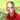January 29, 2019

# Boolean Identities

This post is about a very basic topic in computer programming, which is boolean logic. The main goal here is to avoid formal notation, just write the Identities in an easy to read format.

(1)

``````(\$x || \$y) && \$z
(\$x && \$z) || (\$y && \$z) ``````

(2)

``````(\$x && \$y) || \$z
(\$x || \$z) && (\$y || \$z)``````

(3)

``````\$x === \$y
!\$x XOR \$y
\$x XOR !\$y
true XOR \$x XOR \$y``````

(4)

``````\$x XOR \$y
!\$x === \$y
\$x === !\$y
!(\$x === \$y)``````

(5)

``````\$x XOR \$y
(\$x + \$y) % 2``````

(6)

``````(\$x XOR \$y) && \$z
(\$x && \$z) XOR (\$y && \$z)``````

(7)

``````(\$x && \$y) || \$x
(\$x || \$y) && \$x
\$x``````

(8)

``````\$x XOR \$x
false``````

(9)

``````(\$x XOR \$y) XOR \$x
\$y

(\$x XOR \$y) XOR \$y
\$x``````

(10)

``````!\$x
\$x XOR true``````

(11)

``````!(\$x && \$y)
!\$x || !\$y``````

(12)

``````!(\$x || \$y)
!\$x && !\$y``````

(13)

``````\$x && \$y
!(!\$x || !\$y)
\$x XOR \$y XOR (\$x || \$y)``````

(14)

``````\$x || \$y
!(!\$x && !\$y)
\$x XOR \$y XOR (\$x && \$y)``````

(15)

``````\$x XOR \$y
(\$x || \$y) && !(\$x && \$y)
(\$x && !\$y) || (!\$x && \$y)``````

(16)

``````\$x && false
false

\$x && true
\$x XOR false
\$x``````

# Median operation

Takes three boolean arguments \$x, \$y and \$z. Evaluates to false if most arguments are false, and true if most arguments are true.

``````(\$x && \$y) || (\$y && \$z) || (\$x && \$z)
(\$x || \$y) && (\$y || \$z) && (\$x || \$z)``````

This is also:

• Monotone Boolean function: it can be expressed in terms of && and ||.
• Self-dual monotone formula: && and || can be interchanged.

For real numbers.

``````max(min(\$x, \$y), min(\$y, \$z), min(\$x, \$z))
min(max(\$x, \$y), max(\$y, \$z), max(\$x, \$z))``````

# Truth table

Truth table expresses binary function as a binary string. For example, the median operation is `00010111`:

``````f(0,0,0) = 0
f(0,0,1) = 0
f(0,1,0) = 0
f(0,1,1) = 1
f(1,0,0) = 0
f(1,0,1) = 1
f(1,1,0) = 1
f(1,1,1) = 1``````

# Binary Decision Diagram (BDD)

Binary decision diagram expresses binary function as a diagram. For example, the median operation:

It has the following properties:

• It must be ordered.
• It must be reduced (not waste space).

# References

http://www.cs.utsa.edu/~wagner/knuth/ Donald E. Knuth, The Art of Computer Programming Volume 4Written by Kristjan Siimson who lives and works in Trondheim.